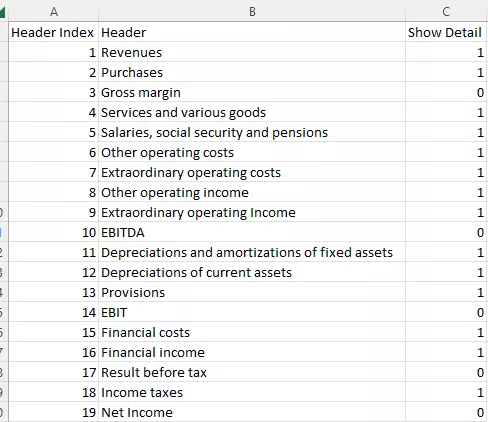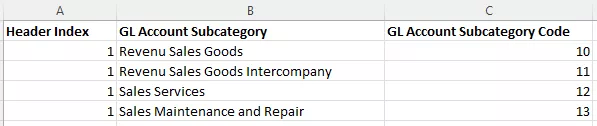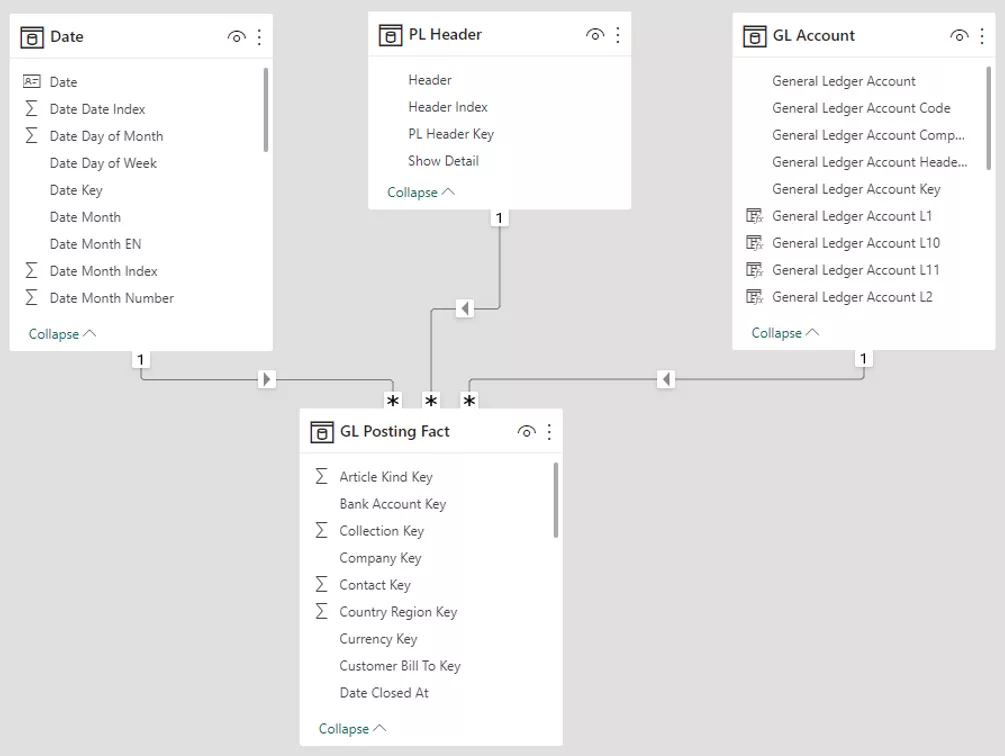# Creating a Dynamic Profit and Loss Statement with Power BI

Financial reporting is an essential aspect of any business as it provides valuable information about the financial performance of the organization. One of the most widely used financial statements is the profit and loss statement, which provides an overview of the company's revenues and expenses over a specified period. Power BI is a powerful data visualization tool that provides the ability to create dynamic and interactive financial reports. In this insight, we will explore the process of creating a profit and loss statement in Power BI

In this insight, we are going to use an Excel to define our P&L structure. The first spreadsheet defines the profit and loss structure and contains three columns: a column with the name, a column with the sort order, and a column called "Show Detail". The sort order column is used to sort the profit and loss in Power BI, and the "Show Detail" column is used to determine whether underlying levels are to be displayed (e.g. revenue) or whether it is a calculated field (e.g. gross margin). The second spreadsheet maps the GL (General Ledger) accounts to the profit and loss structure.

The P&L structure looks something like this:

ImageThe mapping can look like this:

ImageIn this example, we are going to create a relationship between the GL entries and the P&L structure based on GL Account Subcategory Code.

Once the Excel spreadsheets are created, we can load them in our Power BI model or in a data warehouse. The P&L structure we can just load in Power BI does not need any modelling and we will call it PL Header. The P&L mapping sheet we need to join with our table contains all our GL entries (here called GL Posting Fact table). We need to add the “Header Index” columns to this table so we can join it with our PL Header table. The final model should look something like this:

ImageWe then need to sort the Header column from PL Header by “Header Index” to ensure that the P&L is sorted correctly. Next, we will create the DAX measures that will drive our P&L report. The first measure is a measure that sums up  the GL entry amount:

``GL Posting Amount = SUM('GL Posting Fact'[M_Amount])``

The next step is to create a measure that calculates the running total:

``````Running Total (PL) =  CALCULATE (
[GL Posting Amount],
FILTER (
),
'GL Account'[GL Account Income/Balance] = "Income Statement"
)
``````

The running total measure is calculated using the CALCULATE() function, which takes the sum of all previous rows. For example, to calculate the gross margin, we want to take the sum of Revenues and Purchases, and therefore, we take the sum of all rows smaller than or equal to header index 3 (Gross Margin). In a financial system, revenue and costs always have opposite signs, and therefore, this measure will correctly calculate the difference between the two.

Finally, we create the final measure that we will use in our Power BI report:

``````GL Posting Amount (PL) = IF (